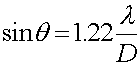The resolving power of a lens

Due to the diffraction pattern caused by a slit, any lens (e.g. a camera lens or the lens in the human eye) will cause a diffraction pattern to occur in the image it makes. As long as the diffraction angle is much less than the angle one wishes to distinguish, this is not a problem. However, the angular resolution of any lens is diffraction limited by the following relation.The diameter of the lens is D, and the wavelength is l. The factor 1.22 is a rather arbitrary choice that is inspired by the shape of a spherical lens pattern which can be written in spherical bessel functions. Since these angles are very small, one can replace sinq with q in the above formula.

Examples     Interference and diffraction's index